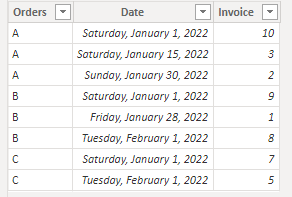cancel
Showing results for
Did you mean:Frequent Visitor

## Create column from another table with sum

Oi.

Tenho uma tabela com informações de pedidos e outra referente aos lançamentos de NFs dos pedidos, gostaria de criar uma coluna na tabela de pedidos com a soma das na tabela de NFs pois existem várias NFs referentes a um pedido.

1 ACCEPTED SOLUTIONCommunity Support

Hi @Greg5 ,

I think your tables should look like as below.

Orders:Invoice:Here are two workarounds to achieve your goal.

1. Create an active relationship between two tables by [Orders] columns.``Sum of Invoice = CALCULATE(SUM(Invoice[Invoice]))``

Result is as below.2. If you don't want to create a relationship or you want to inactive the relationship between two tables, try this code.

``Sum of Invoice = CALCULATE(SUM(Invoice[Invoice]),FILTER(Invoice,Invoice[Orders] = EARLIER(Orders[Orders])))``

Best Regards,
Rico Zhou

If this post helps, then please consider Accept it as the solution to help the other members find it more quickly.Community Support

Hi @Greg5 ,

I think your tables should look like as below.

Orders:Invoice:Here are two workarounds to achieve your goal.

1. Create an active relationship between two tables by [Orders] columns.``Sum of Invoice = CALCULATE(SUM(Invoice[Invoice]))``

Result is as below.2. If you don't want to create a relationship or you want to inactive the relationship between two tables, try this code.

``Sum of Invoice = CALCULATE(SUM(Invoice[Invoice]),FILTER(Invoice,Invoice[Orders] = EARLIER(Orders[Orders])))``

Best Regards,
Rico Zhou

If this post helps, then please consider Accept it as the solution to help the other members find it more quickly.